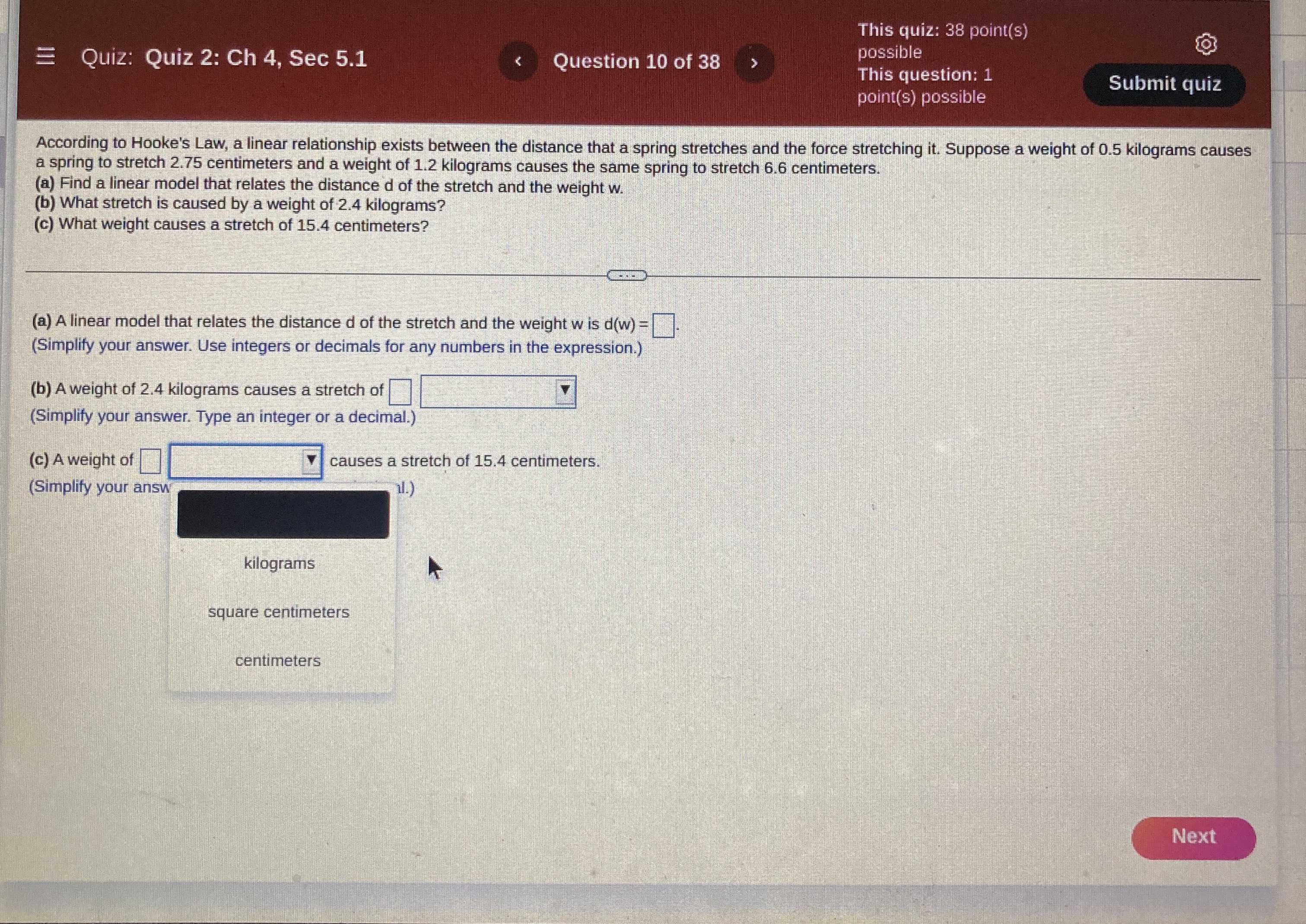### Still have math questions?

Algebra
QuestionAccording to Hooke's Law, a linear relationship exists between the distance that a spring stretches and the force stretching it. Suppose a weight of $$0.5$$ kilograms causes a spring to stretch $$2.75$$ centimeters and a weight of $$1.2$$ kilograms causes the same spring to stretch $$6.6$$ centimeters.

(a) Find a linear model that relates the distance $$d$$ of the stretch and the weight w.

(b) What stretch is caused by a weight of $$2.4$$ kilograms?

(c) What weight causes a stretch of $$15.4$$ centimeters?

a) d= 5.5w

b)13.2 cm

c) 2.8 kilogram

Solution
View full explanation on CameraMath App.